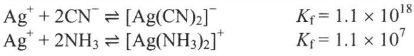# Problem: Silver chloride is relatively insoluble in water (Ksp for AgCl = 1.8 x 10 -10). Given the equation below.Which would you add to dissolve as much AgCl as possible?A) NH3B) KCN

###### Problem Details

Silver chloride is relatively insoluble in water (Ksp for AgCl = 1.8 x 10 -10). Given the equation below.

Which would you add to dissolve as much AgCl as possible?

A) NH3

B) KCNWhat scientific concept do you need to know in order to solve this problem?

Our tutors have indicated that to solve this problem you will need to apply the Formation Constant concept. If you need more Formation Constant practice, you can also practice Formation Constant practice problems.

What is the difficulty of this problem?

Our tutors rated the difficulty ofSilver chloride is relatively insoluble in water (Ksp for Ag...as high difficulty.

How long does this problem take to solve?

Our expert Chemistry tutor, Dasha took 2 minutes and 48 seconds to solve this problem. You can follow their steps in the video explanation above.

What professor is this problem relevant for?

Based on our data, we think this problem is relevant for Professor Korolev's class at UF.